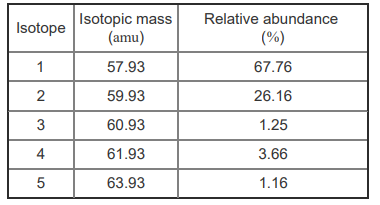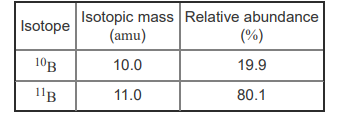# Problem: What is the relative atomic mass of the element that consists of the following isotopes in the indicated natural abundances?Express your answer to three significant figures and include the appropriate units.Most elements occur naturally as a mix of different isotopes. The atomic weight, also called the relative atomic mass, of an element is the weighted average of the isotope masses. In other words, it is an average that takes into account the percentage of each isotope. For example, the two naturally occurring isotopes of boron are given here.The relative atomic mass of boron is calculated as follows: (10.0 × 0.199) + (11.0 × 0.801) = 10.8 amuBecause the heavier isotope is more abundant, the relative atomic mass is closer to 11 amu than it is to 10 amu.

###### FREE Expert Solution
98% (439 ratings)View Complete Written Solution
###### Problem Details

What is the relative atomic mass of the element that consists of the following isotopes in the indicated natural abundances?Express your answer to three significant figures and include the appropriate units.

Most elements occur naturally as a mix of different isotopes. The atomic weight, also called the relative atomic mass, of an element is the weighted average of the isotope masses. In other words, it is an average that takes into account the percentage of each isotope. For example, the two naturally occurring isotopes of boron are given here.The relative atomic mass of boron is calculated as follows:

(10.0 × 0.199) + (11.0 × 0.801) = 10.8 amu

Because the heavier isotope is more abundant, the relative atomic mass is closer to 11 amu than it is to 10 amu.